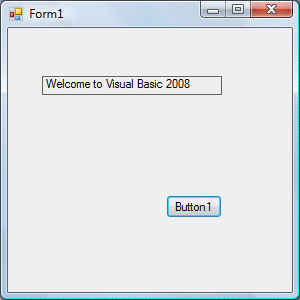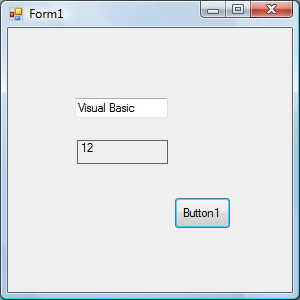# Lesson 8: String Manipulation

String manipulation is an important part of programming because it helps to process data that come in the form of non-numeric types such as name, address, gender, city, book title and more.

### 8.1 String Manipulation Using + and & signs.

Strings can be manipulated using the & sign and the + sign, both perform the string concatenation which means combining two or more smaller strings into larger strings. For example, we can join "Visual" and "Basic" into "Visual Basic" using "Visual"&"Basic" or "Visual "+"Basic", as shown in the example below

#### Example 8.1

Public Class Form1

Private Sub Button1_Click(ByVal sender As System.Object, ByVal e As System.EventArgs) Handles Button1.Click
Dim text1, text2, text3 As String
text1 = "Visual"
text2 = "Basic"
text3 = text1 + text2
Label1.Text = text3

End Sub
End Class

The line  text3=text1+ text2 can be replaced by text3=text1 & text2  and produced the same output. However, if one of the variables is declared as numeric data type, you cannot use the + sign, you can only use the & sign.

Example 8.2

Dim text1, text3 as string

Dim Text2 As Integer

text1 = "Visual"

text2=22

text3=text1+text2

Label1.Text = text3

This code will produce an error because of data mismatch.However, using & instead of + will be all right.

Dim text1, text3 as string

Dim Text2 As Integer

text1 = "Visual"

text2=22

text3=text1 & text2

Label1.Text = text3

You can combine more than two strings to form a larger strings, like the following example:

Public Class Form1

Private Sub Button1_Click(ByVal sender As System.Object, ByVal e As System.EventArgs) Handles Button1.Click
Dim text1, text2, text3, text4, text5, text6 As String

text1 = "Welcome"
text2 = " to"
text3 = " Visual"
text4 = " Basic"
text5 = " 2008"
text6 = text1 + text2 + text3+text4+Text5
Label1.Text = text6

End Sub
End Class

Running the above program will produce the screen shotas shown in Figure 8.1.### 8.2 String Manipulation Using VB2008 Built-in Functions

A function is similar to a normal procedure but the main purpose of the function is to accept a  certain input and return a value which is passed on to the main program to finish the execution.There are numerous string manipulation functions built into VB2008 but I will only discuss a few here and will explain the rest of them in later lessons.

#### 8.2 (a) The Len Function

The length function returns an integer value which is the length of a phrase or a sentence, including the empty spaces. The format is

`Len(“Phrase”)`

For example,

Len (Visual Basic)= 12 and Len (welcome to VB tutorial) = 22

#### Example 8.3

``` Public Class Form1
Private Sub Button1_Click(ByVal sender As System.Object, ByVal e As System.EventArgs) Handles Button1.Click
Label1.Text =
Len(TextBox1.Text)
End Sub
End Class
```

The output is shown in Figure 8.2#### 8.2(b) The Right  Function

The Right function extracts the right portion of a phrase. The format for Visual Basic 6 is

Right (“Phrase”, n)

Where n is the starting position from the right of the phase where the portion of the phrase is going to be extracted.  For example,

Right(“Visual Basic”, 4) = asic

However, this format is not applicable in VB2008. In VB2008, we need use the following format

Microsoft.VisualBasic.Right("Phrase",n)

Example 8.3

Private Sub Button1_Click(ByVal sender As System.Object, ByVal e As System.EventArgs) Handles Button1.Click
Dim text1 As String

text1 = TextBox1.Text

Label1.Text = Microsoft.VisualBasic.Right(text1, 4)

End Sub

The above program will return four right most characters of the phrase entered into the textbox.

The Output#### Figure 8.3

*The reason of using the full reference is because many objects have the Right properties so using Right on its own will make it ambiguous to VB2008.

#### 8.2(c)The Left Function

The Left function extract the left portion of a phrase. The format is

Microsoft.VisualBasic.Left("Phrase",n)

Where n is the starting position from the left of the phase where the portion of the phrase is going to be extracted.  For example,

Microsoft.VisualBasic.Left (“Visual Basic”, 4) = Visu  .

I Other functions will be discussed in future lessons.